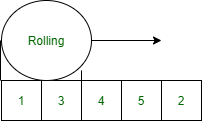GeeksforGeeks App
Open AppBrowser
Continue

# Implementing Rabin Karp Algorithm Using Rolling Hash in Java

There are so many pattern searching algorithms for the string. KMP algorithm, Z algorithm Rabin Karp algorithm, etc these algorithms are the optimization of Naive Pattern searching Algorithm.

Naive Pattern Searching Algorithm:

```Input   :  "AABACACAACAC"
Pattern :  "CAC"
Output  :  [4,9]

AABACACAACAC```

Implementation:

## Java

 `// Java Program to Search for a Pattern String in the Input``// String returning the indices` `// Importing input java classes``import` `java.io.*;` `// Main class``public` `class` `GFG {` `    ``// Method 1``    ``// To find pattern in the string``    ``static` `void` `search(String s, String pattern)``    ``{``        ``// Iterating over the string in which to be searched``        ``// for using the length() method``        ``for` `(``int` `i = ``0``; i <= s.length() - pattern.length();``             ``++i) {` `            ``int` `check = ``1``;` `            ``// Iterating over the string for which is to be``            ``// searched using the length() method``            ``for` `(``int` `j = ``0``; j < pattern.length(); ++j) {` `                ``// Now, checking the elements of pattern``                ``// with the given string using the charAt()``                ``// method``                ``if` `(s.charAt(i + j) != pattern.charAt(j)) {` `                    ``// Setting check to zero as pattern is``                    ``// not detected here``                    ``check = ``0``;` `                    ``// Break statement to hault``                    ``// execution of code``                    ``break``;``                ``}``            ``}` `            ``// Now if the check remains same as declared``            ``// then pattern is detected at least once``            ``if` `(check == ``1``) {` `                ``// Printing the position(index) of the``                ``// pattern string in the input string``                ``System.out.print(i + ``" , "``);``            ``}``        ``}``    ``}` `    ``// Method 2``    ``// Main driver method``    ``public` `static` `void` `main(String[] args)``    ``{``        ``// Given custom input string``        ``String s = ``"AABACACAACAC"``;` `        ``// Pattern to be looked after in``        ``// the above input string``        ``String pattern = ``"CAC"``;` `        ``// Display message for interpreting the indices``        ``// ai where if the pattern exists``        ``System.out.print(``            ``"Pattern is found at the indices : "``);` `        ``// Calling the above search() method``        ``// in the main() method``        ``search(s, pattern);``    ``}``}`

Output

`Pattern is found at the indices : 4 , 9 , `

Output explanation:

The above algorithm for pattern searching is the basic algorithm the worst as the average time complexity of this algorithm is O(n×m) where n is the pattern and m is the given string.

How can we reduce the complexity of this algorithm?

It is possible with the help of rolling hash. Rabin Karp algorithm is one of the optimized algorithms of the naive algorithm that performs searching by rolling over the string and search the pattern.Illustration:

```Input:  txt[] = "THIS IS A TEST TEXT"
pat[] = "TEST"
Output: Pattern found at index 10

pat[] =  "AABA"
Output: Pattern found at index 0
Pattern found at index 9
Pattern found at index 12```

Procedure:

• Calculate the hash value of the pattern (By creating your own hash function or equation to determining an individual hash value for every character)
• Iterate over the string check the hash value of every substring generated of the size of the pattern if matched check every character of the pattern as well as String if all matched print the starting index of the string.
• If not matched shift to the next character by skipping the first character and adding the hash value of the next character that we don’t calculate the hash value of the next substring in the string only we slide the window skip the first character and add the last character in the window by calculating its hash value i.e Rolling hash.

Implementation:

Simple Rolling algorithm assuming the pattern of length 2

1. Initialize temp=4(1+3)
2. Roll the hash value to the next element.
3. Iterate the loop ‘i’ <= Array.length-pattern.length
4. Remove the first element from the temp variable and add next element in the temp variable. temp = temp-Array[i]+Array[i.pattern.length()]

## Java

 `// Java Program to illustrating Simple Rolling Hashing` `// Importing input output classes``import` `java.io.*;` `// Main class``class` `GFG {` `    ``// Method 1``    ``// To search the pattern``    ``static` `void` `search(String S, String pattern)``    ``{``        ``// Declaring and initializing the hash values``        ``int` `hash1 = ``0``;``        ``int` `hash2 = ``0``;` `        ``// Iterating over the pattern string to be matched``        ``// over``        ``for` `(``int` `i = ``0``; i < pattern.length(); ++i) {` `            ``// Storing the hash value of the pattern``            ``hash1 += pattern.charAt(i) - ``'A'``;` `            ``// Storing First hash value of the string``            ``hash2 += S.charAt(i) - ``'A'``;``        ``}` `        ``// Initially declaring with zero``        ``int` `j = ``0``;` `        ``// Iterating over the pattern string to checkout``        ``// hash values``        ``for` `(``int` `i = ``0``; i <= S.length() - pattern.length();``             ``++i) {` `            ``// Checking the hash value``            ``if` `(hash2 == hash1) {` `                ``// Checking the value``                ``for` `(j = ``0``; j < pattern.length(); ++j) {` `                    ``// Checking for detection of pattern in a``                    ``// pattern``                    ``if` `(pattern.charAt(j)``                        ``!= S.charAt(i + j)) {` `                        ``// Break statement to hault the``                        ``// execution of program as no``                        ``// pattern found``                        ``break``;``                    ``}``                ``}``            ``}` `            ``// If execution is not stopped means``            ``// pattern(sub-string) is present` `            ``// So now simply detecting for one or more``            ``// occurrences inbetween pattern string using the``            ``// length() method``            ``if` `(j == pattern.length()) {` `                ``// Pattern is detected so printing the index``                ``System.out.println(i);``            ``}``            ``// for last case of loop, have to check,``            ``// otherwise,``            ``// S.charAt(i + pattern.length()) below will``            ``// throw error``            ``if` `(i == S.length() - pattern.length())``                ``break``;` `            ``// Roll the hash value over the string detected``            ``hash2 = (``int``)((hash2) - (S.charAt(i) - ``'A'``))``                    ``+ S.charAt(i + pattern.length()) - ``'A'``;``        ``}``    ``}` `    ``// Method 2``    ``// Main driver method``    ``public` `static` `void` `main(String[] args)``    ``{` `        ``// Input string to be traversed``        ``String S = ``"AABAACAADAABAABA"``;` `        ``// Pattern string to be checked``        ``String pattern = ``"AABA"``;` `        ``// Calling the above search() method(Method1)``        ``// in the main() method``        ``search(S, pattern);``    ``}``}`

Output

```0
9
12```

My Personal Notes arrow_drop_up